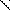# MINLPLib

### A Library of Mixed-Integer and Continuous Nonlinear Programming Instances

#### Instance: ball_mk4_05

```A simple MINLP with a feasible set described by a ball.
The basic model over which these variations are made is:
min  sum_i=1^n x_i
s.t. sum_i=1^n (x_i - 0.5)^2 <= (n-1)/4
x integer between -1 and 1.
Obvisouly, this problem is infeasible and has no solution.
It can be shown that any outer-approximation based method will need 2^n linear inequalities to show infeasibility, see reference.
In this instance, the ball is an empty ellipse and the quadratic form is not diagonal.```
 Formatsⓘ ams gms lp mod nl osil pip Primal Boundsⓘ Dual Boundsⓘ 36.00054285 (ALPHAECP)5.99788343 (ANTIGONE)inf (BARON)inf (BONMIN)25220.00000000 (COUENNE)inf (LINDO)inf (SCIP) Referencesⓘ Hijazi, Hassan, Bonami, Pierre, and Ouorou, Adam, An Outer-Inner Approximation for Separable Mixed-Integer Nonlinear Programs, INFORMS Journal on Computing, 26:1, 2014, 31-44. Sourceⓘ Pierre Bonami Applicationⓘ Geometry Added to libraryⓘ 11 Sep 2017 Problem typeⓘ IQCP #Variablesⓘ 10 #Binary Variablesⓘ 0 #Integer Variablesⓘ 10 #Nonlinear Variablesⓘ 10 #Nonlinear Binary Variablesⓘ 0 #Nonlinear Integer Variablesⓘ 10 Objective Senseⓘ min Objective typeⓘ linear Objective curvatureⓘ linear #Nonzeros in Objectiveⓘ 10 #Nonlinear Nonzeros in Objectiveⓘ 0 #Constraintsⓘ 1 #Linear Constraintsⓘ 0 #Quadratic Constraintsⓘ 1 #Polynomial Constraintsⓘ 0 #Signomial Constraintsⓘ 0 #General Nonlinear Constraintsⓘ 0 Operands in Gen. Nonlin. Functionsⓘ Constraints curvatureⓘ convex #Nonzeros in Jacobianⓘ 10 #Nonlinear Nonzeros in Jacobianⓘ 10 #Nonzeros in (Upper-Left) Hessian of Lagrangianⓘ 20 #Nonzeros in Diagonal of Hessian of Lagrangianⓘ 10 #Blocks in Hessian of Lagrangianⓘ 5 Minimal blocksize in Hessian of Lagrangianⓘ 2 Maximal blocksize in Hessian of Lagrangianⓘ 2 Average blocksize in Hessian of Lagrangianⓘ 2.0 #Semicontinuitiesⓘ 0 #Nonlinear Semicontinuitiesⓘ 0 #SOS type 1ⓘ 0 #SOS type 2ⓘ 0 Infeasibility of initial pointⓘ 1 Sparsity JacobianⓘSparsity Hessian of Lagrangianⓘ```\$offlisting
*
*  Equation counts
*      Total        E        G        L        N        X        C        B
*          2        1        0        1        0        0        0        0
*
*  Variable counts
*                   x        b        i      s1s      s2s       sc       si
*      Total     cont   binary  integer     sos1     sos2    scont     sint
*         11        1        0       10        0        0        0        0
*  FX      0
*
*  Nonzero counts
*      Total    const       NL      DLL
*         21       11       10        0
*
*  Solve m using MINLP minimizing objvar;

Variables  objvar,i2,i3,i4,i5,i6,i7,i8,i9,i10,i11;

Integer Variables  i2,i3,i4,i5,i6,i7,i8,i9,i10,i11;

Equations  e1,e2;

e1..    objvar - 9*i2 - 8*i3 - 7*i4 - 6*i5 - 5*i6 - 4*i7 - 3*i8 - 2*i9 - i10
- 10*i11 =E= 0;

e2.. 100*sqr(i10) - 98*i10 + 100*sqr(i9) - 98*i9 + 100*sqr(i8) - 98*i8 + 100*
sqr(i7) - 98*i7 + 100*sqr(i6) - 98*i6 + 100*sqr(i5) - 98*i5 + 100*sqr(i4)
- 98*i4 + 100*sqr(i3) - 98*i3 + 100*sqr(i2) - 98*i2 + 100*sqr(i11) - 98*
i11 - 2*i10*i9 - 2*i10*i9 - 2*i8*i7 - 2*i8*i7 - 2*i6*i5 - 2*i6*i5 - 2*i4*
i3 - 2*i4*i3 - 2*i2*i11 - 2*i2*i11 =L= -1;

* set non-default bounds
i2.lo = -100;
i3.lo = -100;
i4.lo = -100;
i5.lo = -100;
i6.lo = -100;
i7.lo = -100;
i8.lo = -100;
i9.lo = -100;
i10.lo = -100;
i11.lo = -100;

Model m / all /;

m.limrow=0; m.limcol=0;
m.tolproj=0.0;
\$if gamsversion 242 option intvarup = 0;

\$if NOT '%gams.u1%' == '' \$include '%gams.u1%'

\$if not set MINLP \$set MINLP MINLP
Solve m using %MINLP% minimizing objvar;

```

Last updated: 2019-02-14 Git hash: a71254dc